# ISEE Middle Level Math : Algebraic Concepts

## Example Questions

### Example Question #1241 : Hspt Mathematics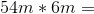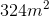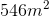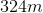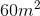Explanation:

Multiply the constants and add an exponent to the variable totaling the number of variables in the equation: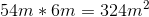Answer:### Example Question #1242 : Hspt Mathematics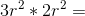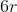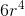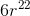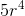Explanation:

Multiply the whole numbers and add the exponents: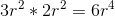Answer:### Example Question #1243 : Hspt Mathematics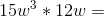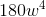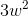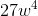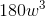Explanation:

Multiply the whole numbers and add the exponents: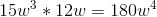Answer:### Example Question #1244 : Hspt Mathematics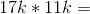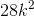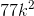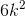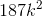Explanation:

Multiply the whole numbers and add the exponents: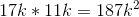### Example Question #1251 : Hspt Mathematics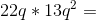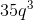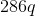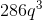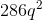Explanation:

Multiply the whole numbers and add the exponents: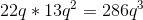### Example Question #1252 : Hspt Mathematics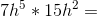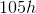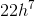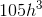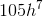Explanation:

Multiply the whole numbers and add the exponents: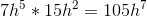### Example Question #1253 : Hspt Mathematics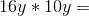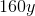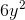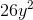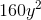Explanation:

Multiply the whole numbers and add the exponents: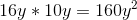Answer:### Example Question #1254 : Hspt Mathematics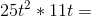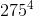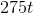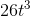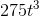Explanation:

Multiply the whole numbers and add the exponents: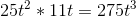Answer:### Example Question #1255 : Hspt Mathematics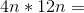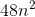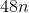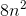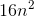Explanation:

Multiply the whole numbers and add the exponents: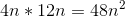Answer:### Example Question #1256 : Hspt Mathematics

Simplify the expression: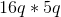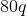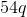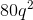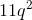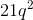Explanation:

The commutative property lets us reorganize the expression.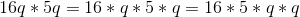By separating the whole numbers and the variables, we can easily multiply.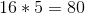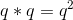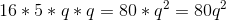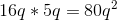Answer:### All ISEE Middle Level Math Resources